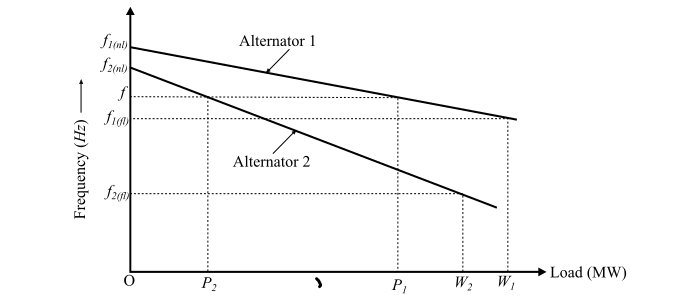# Load Sharing by Two Alternators in Parallel Operation

Consider two synchronous generators or alternators are operating in parallel and their load-frequency characteristics are shown in the figure.Let

• $𝑓_{1(𝑛𝑙)}$ = No load frequency of alternator 1

• $𝑓_{2(𝑛𝑙)}$ = No load frequency of alternator 2

• $𝑓_{1(𝑓𝑙)}$ = Full load frequency of alternator 1

• $𝑓_{2(𝑓𝑙)}$ = Full load frequency of alternator 2

• 𝑓 = Common operating frequency when the two alternators are operating in parallel

• $𝑊_{1}$ = Full load power rating of alternator 1

• $𝑊_{2}$ = Full load power rating of alternator 2

• $𝑃_{1}$ = Power shared by alternator 1

• $𝑃_{2}$ = Power shared by alternator 2

• 𝑃 = Total power delivered by the two alternators

## For Alternator 1:

$$\mathrm{Drop\:in\:frequency\:from\:no\:load\:to \:full\:load = 𝑓_{1(𝑛𝑙)} − 𝑓_{1(𝑓𝑙)}}$$

$$\mathrm{Drop\:in\:frequency\:per\:unit\:power \:rating =\frac{𝑓_{1(𝑛𝑙)}− 𝑓_{1(𝑓𝑙)}}{𝑊_{1}}}$$

$$\mathrm{∴\:Drop\:in\:frequency\:for\:a\:load\:of \:power\:𝑃_{1} =\frac{𝑓_{1(𝑛𝑙)} − 𝑓_{1(𝑓𝑙)}}{𝑊_{1}}× 𝑃_{1} … (1)}$$

Therefore, the operating frequency of the alternator 1 would be,

$$\mathrm{𝑓_{1} = No\:load\:frequency − Drop\:in\: frequency}$$

$$\mathrm{\Rightarrow\:𝑓_{1} = 𝑓_{1(𝑛𝑙)} − \left( \frac{𝑓_{1(𝑛𝑙)} − 𝑓_{1(𝑓𝑙)}}{𝑊_{1}}× 𝑃_{1}\right)… (2)}$$

## For Alternator 2:

$$\mathrm{Drop\:in\:frequency\:from\:no\:load\:to \:full\:load = 𝑓_{2(𝑛𝑙)} − 𝑓_{2(𝑓𝑙)}}$$

$$\mathrm{Drop\:in\:frequency\:per\:unit\:power \:rating =\frac{𝑓_{2(𝑛𝑙)} − 𝑓_{2(𝑓𝑙)}}{𝑊_{2}}}$$

$$\mathrm{∴\:Drop\:in\:frequency\:for\:a\:load\:of\:power\:𝑃_{2} =\frac{𝑓_{2(𝑛𝑙)} − 𝑓_{2(𝑓𝑙)}}{𝑊_{2}}× 𝑃_{2}… (3)}$$

Therefore, the operating frequency of the alternator 2 would be,

$$\mathrm{𝑓_{2} = No\:load\:frequency − Drop\:in\: frequency}$$

$$\mathrm{\Rightarrow\:𝑓_{2} = 𝑓_{2(𝑛𝑙)} − \left(\frac{𝑓_{2(𝑛𝑙)} − 𝑓_{2(𝑓𝑙)}}{𝑊_{2}}× 𝑃_{2} \right)… (4)}$$

For parallel operation, both the alternators must operate at the same frequency.Thus, from Eqns. (2) and (4), we have,

$$\mathrm{𝑓_{1} = 𝑓_{2} = 𝑓}$$

$$\mathrm{∴\:𝑓 = 𝑓_{1(𝑛𝑙)} −\left( \frac{𝑓_{1(𝑛𝑙)} − 𝑓_{1(𝑓𝑙)}}{𝑊_{1}}× 𝑃_{1} \right)}$$

$$\mathrm{= 𝑓_{2(𝑛𝑙)} −\left(\frac{𝑓_{2(𝑛𝑙)} − 𝑓_{2(𝑓𝑙)}}{𝑊_{2}}× 𝑃_{2} \right)… (5)}$$

Also, the total power delivered by the two alternators is

$$\mathrm{𝑃 = 𝑃_{1 }+ 𝑃_{2} … (6)}$$

Hence, the power shared by the two alternator 𝑃1, 𝑃2 and the common operating frequency (f) of the system can be determined using the eqns. (5) and (6).

## Numerical Example

Two station alternators A and B operate in parallel. The Station capacity of A is 30 MW and that of B is 60 MW. The full-load speed regulation of station A is 4% and full-load speed regulation of B is 4.5%. Calculate the load sharing if the connected load is 60 MW. No-load frequency is 50 Hz.

Solution

Let

• $𝑃_{𝐴}$ = load shared by alternator 𝐴 in MW

• $𝑃_{𝐵}$ = load shared by alternator 𝐵 in MW

$$\mathrm{∴\:𝑃_{𝐴} + 𝑃_{𝐵} = 60\:MW … (1)}$$

Original frequency of the system at no-load is 𝑓𝑛𝑙 = 50 Hz

### Alternator A:

For a load of 30 MW,

$$\mathrm{the\:drop\:in\:frequency = 4\%\:of\:𝑓_{𝑛𝑙 }=\frac{4}{100}\times 50 = 2\:Hz}$$

For a load of 1 MW,

$$\mathrm{the\:drop\:in\:frequency =\frac{2}{30}= 0.067\:Hz}$$

∴ For a load of 𝑃𝐴 MW,

$$\mathrm{the\:drop\:in\:frequency =\frac{2}{30}\times 𝑃_{𝐴}=0.067𝑃_{𝐴}\:Hz}$$

Hence, the operating frequency of alternator A is,

$$\mathrm{𝑓_{𝐴} = 𝑓_{𝑛𝑙} − (drop\:in\:frequency)}$$

$$\mathrm{\Rightarrow\:𝑓_{𝐴} = 50 − 0.067𝑃_{𝐴} … (2)}$$

### Alternator B:

Similarly, the operating frequency of alternator B is given by,

$$\mathrm{𝑓_{𝐵} = 𝑓_{𝑛𝑙} − (drop\:in\:frequency)}$$

$$\mathrm{\Rightarrow\:𝑓_{𝐵} = 50 − \left( \frac{4.5}{100} \times 50\right)\frac{𝑃_{𝐵}}{60}}$$

$$\mathrm{\Rightarrow\:𝑓_{𝐵} = 50 − 0.0375\:𝑃_{𝐵} … (3)}$$

Since for parallel operation both the alternators must operate at the same frequency, we have,

$$\mathrm{𝑓_{𝐴} = 𝑓_{𝐵}}$$

$$\mathrm{\Rightarrow\:50 − 0.067𝑃_{𝐴} = 50 − 0.0375𝑃_{𝐵}}$$

$$\mathrm{\Rightarrow\:134𝑃_{𝐴} = 75𝑃_{𝐵}}$$

$$\mathrm{\Rightarrow\:𝑃_{𝐴} =\frac{75}{134}𝑃_{𝐵} … (4)}$$

And,

$$\mathrm{𝑃_{𝐵} =\frac{134}{75}𝑃_{𝐴} … (5)}$$

From Eqns. (1), (4) and (5), we get,

$$\mathrm{\frac{75}{134}𝑃_{𝐵} + 𝑃_{𝐵} = 60\:MW}$$

$$\mathrm{\Rightarrow\:𝑃_{𝐵 }= 38.71\:MW}$$

$$\mathrm{𝑃_{𝐴} = 60 − 38.71 = 21.29\:MW}$$